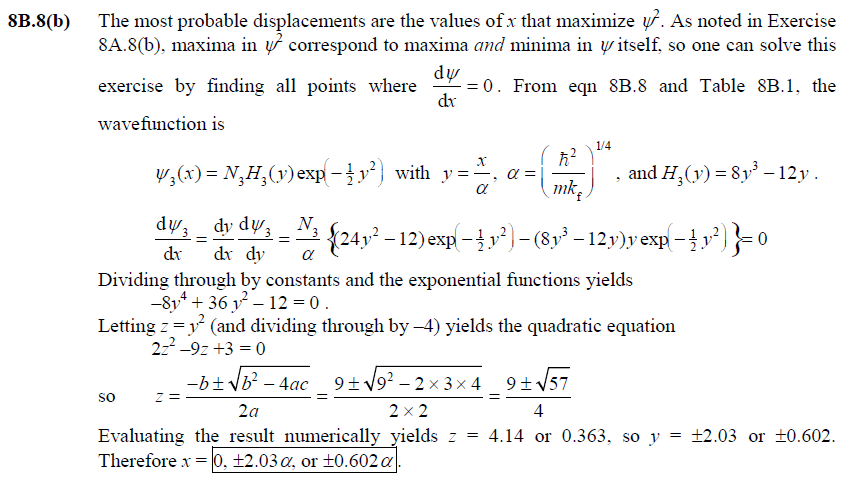# CHAPTER 8B Problem Solving Flashcards

Set Details Share
created 1 year ago by miaprimrosee
196 views
Subjects:
phychem3
Page to share:
Embed this setcancel
COPY
code changes based on your size selection
Size:
X

1

8B.2 In what ways does the quantum mechanical description of a harmonic oscillator merge with its classical description at high quantum numbers?

The correspondence principle (mentioned in Topic 8A.2(b)) states that in the limit of very large quantum numbers quantum mechanics merges with classical mechanics. The harmonic oscillator provides an example. A classical harmonic oscillator’s range of motion is restricted by classical turning points, which are determined by the oscillator’s total energy; that energy can take on any real positive value. By contrast, a quantum harmonic oscillator can tunnel past classical turning points into the classically forbidden region with a
non-zero probability. The total energy of a quantum harmonic oscillator is quantized; not every real positive value is allowed. At high quantum numbers, the probability of tunneling
beyond the classical turning points falls (approaching the zero probability of classical harmonic oscillators). Furthermore, the most likely place to find the oscillator is near the classical turning points. (This is true of the classical oscillator as well: because the speed of
the oscillator vanishes at the turning points, the oscillator spends more time near the turning points than elsewhere in its range.) See Figure 8B.7 of the main text, particularly for v = 18,
to see the probability distribution for large v approach the classical picture. Finally, although the spacing between discrete allowed energy levels is the same size at large quantum numbers as at small ones, that spacing is a smaller fraction of total energy at large quantum numbers; in that sense, the allowed energy levels are more nearly continuous at
large quantum numbers than small.

2

8B.1(b) Calculate the zero-point energy of a harmonic oscillator consisting of a particle of mass 5.16 × 10−26 kg and force constant 285 N m−1.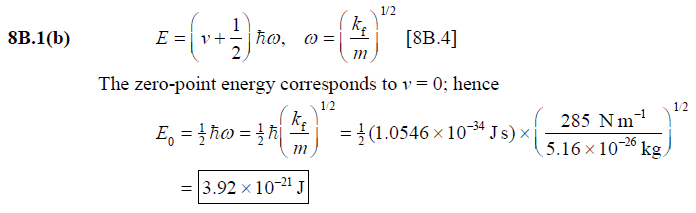3

8B.2(b) For a certain harmonic oscillator of effective mass 2.88 × 10−25 kg, the difference in adjacent energy levels is 3.17 zJ. Calculate the force constant of
the oscillator.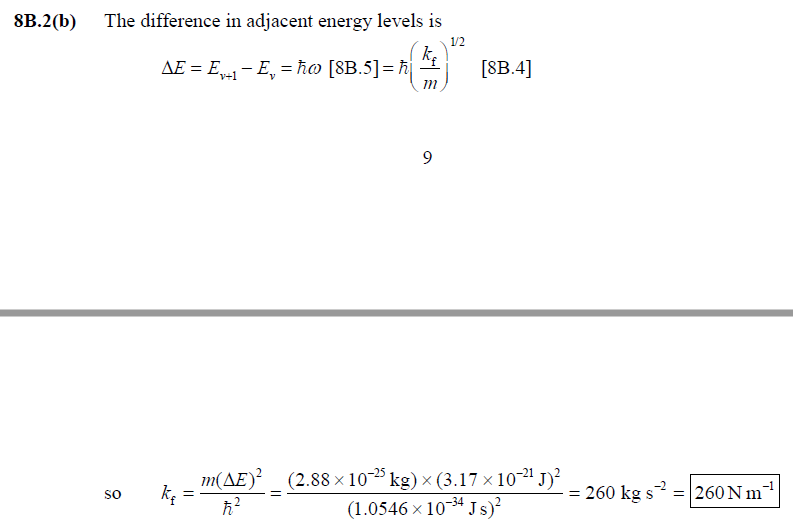4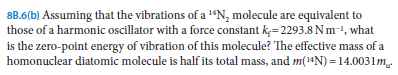8B.6(b)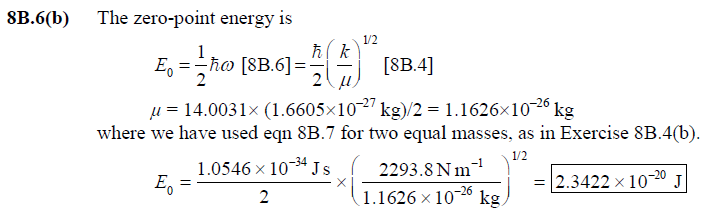5

8B.7(b) Locate the nodes of the harmonic oscillator wavefunction with v = 5.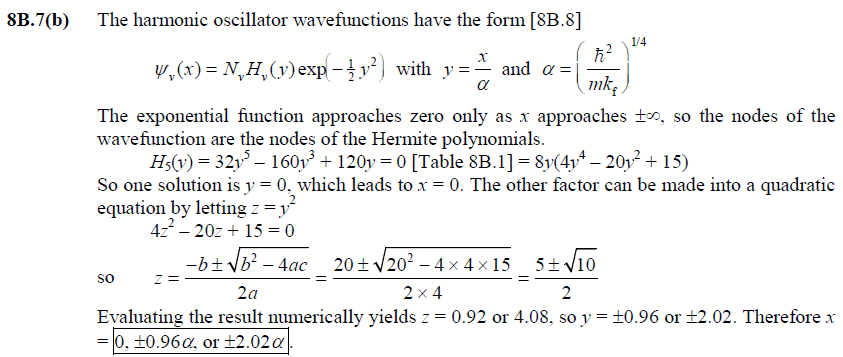6

8B.8(b) What are the most probable displacements of a harmonic oscillator with v = 3?